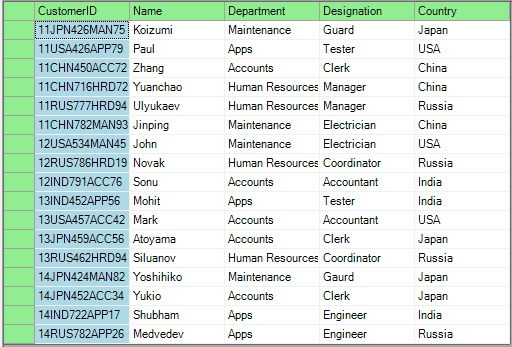Alphanumeric format is one of the most widely used formats for representing data. They are used nearly everywhere, from Employee IDs to check/demand draft numbers in banks. Sorting of alphanumeric data is not a hard nut to crack till the data is simple (say “AB345”). C1FlexGrid's built-in sorting engine allows you to sort this type of data in the order in which alphabets and numerals appears. For example, the values “AB3455”, “AA746” and”AB3422” are first sorted according to the alphabets and then by numerals. But what if the alphanumeric values are complex (say, ”13USA457ACC42”) which essentially means that they may contain numerals and alphabets in an anonymous order and the requirement is to sort them according to the numerals in them?

Sounds difficult ???

No, it's really not.

Before moving further to the implementation, let us take a look at our final output.## Implementation

In the BeforeSort event of C1FlexGrid, create a temporary table and populate it only with the numerical part of the alphanumeric field. To accomplish the same, we can use regular expressions as follows:

``````

resultString = System.Text.RegularExpressions.Regex.Replace(subjectString, @"\\D", "");          //For taking out numerical data

``````

Now, sort this temporary table using any of the sorting algorithms (Bubble sort used in this particular case) and apply same swapping order in the main table (to which the grid is bound) as well.

``````

for (int i = 0; i < tempNumTable.Rows.Count ; i++)
{
for (int j = 0; j < tempNumTable.Rows.Count - 1 - i ; j++)                                                     {  if (Convert.ToInt32(tempNumTable.Rows[j].ItemArray) > Convert.ToInt32(tempNumTable.Rows[j + 1].ItemArray))
{
temp = Convert.ToInt32(tempNumTable.Rows[j + 1]);
tempNumTable.Rows[j + 1] = tempNumTable.Rows[j];
tempNumTable.Rows[j] = temp;
tempRow1 = tempTable.NewRow();
//Temporary Rows used for swapping
tempRow2 = tempTable.NewRow();
tempRow1.ItemArray = tempTable.Rows[j].ItemArray;
tempRow2.ItemArray = tempTable.Rows[j+1].ItemArray;
tempTable.Rows.RemoveAt(j);
tempTable.Rows.RemoveAt(j);
tempTable.Rows.InsertAt(tempRow2, j);
tempTable.Rows.InsertAt(tempRow1, j + 1);

}

}

}

``````

Please make sure to cancel the BeforeSort and AfterSort events in order to suppress the default sorting of C1FlexGrid.

``````

e.Cancel = true;

``````

The best thing about this sorting technique is that it can be used to sort alphanumeric data for nearly all types of grids :) FlexGrid Sorting - C# FlexGrid Sorting - VB